Courses
Courses for Kids
Free study material
Free LIVE classes
More

# Entropy of Different Process for JEELIVE
Join Vedantu’s FREE Mastercalss

## What is Entropy?

Entropy is a measure of a system's unpredictability or disorder, according to its definition. In 1850, a German physicist, Rudolf Clausius first proposed this idea. We do not consider a system's infinitesimal parts from a thermodynamics perspective on entropy of different processes. The behaviour of a system is instead described in terms of thermodynamic parameters such as temperature, pressure, entropy, and heat capacity using the concept of entropy of different processes.

Statistics also explain the concept of entropy. It is used to describe the atomic movements that take place in a system. Therefore, it is a molecular disorder measured according to the statistical definition. Additionally, compared to a gas, a solid has a higher entropy (closely packed particles) (particles are free to move). The second law of thermodynamics developed the idea of entropy of different processes.

## Entropy in Reversible and Irreversible Process

• In this situation, the second law of thermodynamics states that depending on the internal process, the container's entropy must either rise or stay the same at the end of a reversible operation.

• Entropy can never be negative for the universe, according to this argument as well.

• It also states that in a reversible process, the entropy of the universe is constant, whereas in an irreversible process, the universe becomes more entropic as heat is transferred from a warm to a chilly thing.

## Change in Entropy

Entropy change is a phenomenon that measures the evolution of randomness or disorder in a thermodynamic system. It has to do with how heat or enthalpy is converted during work. More unpredictability in a thermodynamic system indicates high entropy.

To calculate the entropy we will use the entropy formula thermodynamically.

The formula of entropy change  is written as:

$\Delta S=\dfrac{q}{T}$

Where $q$ refers to the heat that transfers energy during a process of change in the entropy equation, and $T$ refers to the temperature in Kelvin. Entropy, measured in SI units is joules per Kelvin (J/K).

## Entropy in Different Thermodynamic Process

1. Isobaric Process: The process in which there is no change in pressure is known as isobaric process. Think about a ideal gas under a constant pressure and its temperature changes from ${{T}_{1}}$ to ${{T}_{2}}$ and entropy changes from ${{S}_{1}}$ to ${{S}_{2}}$.

Then,

$Q={{C}_{p}}({{T}_{2}}-{{T}_{1}})$

Differentiate to find a small increase in heat, where the dQ of the ideal gas when temperature rises, is dT.

$dQ={{C}_{p}}dT$

Dividing both sides by T, we get

$dQ/T=m~{{C}_{p}}dT/T$

$\Rightarrow dS=m~{{C}_{p}}dT/T$

Integrating both sides, we get

$\int\limits_{{{S}_{1}}}^{{{S}_{2}}}{dS}=m~{{C}_{p}}\int\limits_{{{T}_{1}}}^{{{T}_{2}}}{dT/T}$

$\Rightarrow {{S}_{2}}-{{S}_{1}}=m~{{C}_{p}}\ln ({{T}_{2}}/{{T}_{1}})$

$\Rightarrow \Delta {{S}_{p}}=m~{{C}_{p}}\ln ({{T}_{2}}/{{T}_{1}})$

As a result, an ideal gas's increasing temperature at constant pressure is accompanied by an increase in entropy.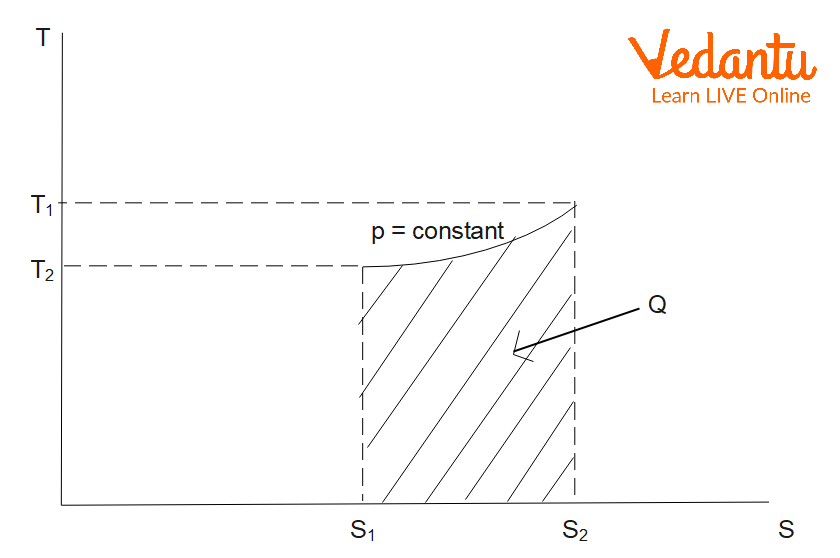Graph of Isobaric Process

2. Isochoric Process: The process in which there is no change in volume is known as isochoric process. Think about a ideal gas at constant volume and its temperature changes from ${{T}_{1}}$ to ${{T}_{2}}$ and entropy changes from ${{S}_{1}}$ to ${{S}_{2}}$.

Then

$Q=m~{{C}_{V}}({{T}_{2}}-{{T}_{1}})$

On differentiating, we get

$dQ=m~{{C}_{V}}dT$

Dividing both sides by T, we get

$dQ/T=m~{{C}_{V}}dT/T$

$\Rightarrow dS=m~{{C}_{V}}dT/T$

Integrating both sides,

$\int\limits_{{{S}_{1}}}^{{{S}_{2}}}{dS}=m~{{C}_{V}}\int\limits_{{{T}_{1}}}^{{{T}_{2}}}{dT/T}$

$\Rightarrow {{S}_{2}}-{{S}_{1}}=m~{{C}_{V}}\ln ({{T}_{2}}/{{T}_{1}})$

$\Rightarrow \Delta {{S}_{P}}=m~{{C}_{V}}\ln ({{T}_{2}}/{{T}_{1}})$

As a result, an ideal gas's entropy increases along with its temperature when the ideal gas' volume remains constant.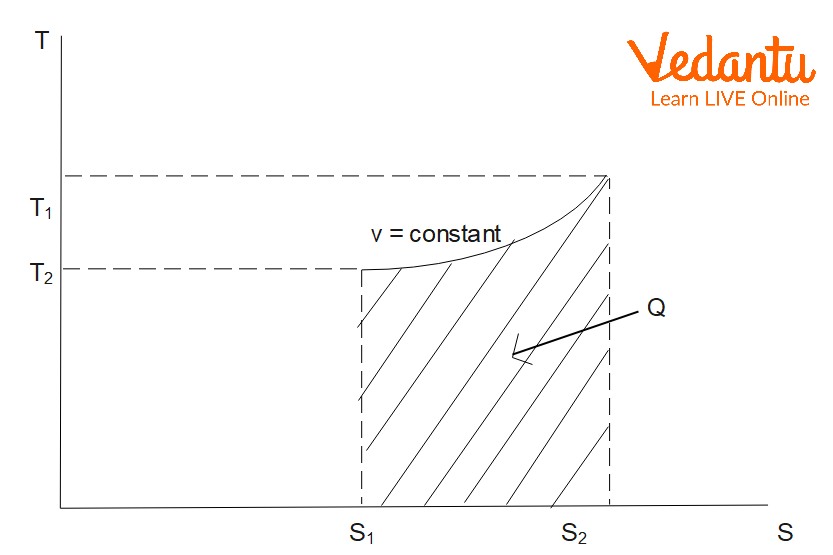Graph of Isochoric Process

3. Isothermal Process: Entropy change in isothermal process changes from ${{S}_{1}}$ to ${{S}_{2}}$ when gas absorbs heat during expansion. The area under the curve, which reflects the work done during expansion, is what determines how much heat the gas absorbs.

In other words, $Q=W$.

But

$Q={{S}_{2}}\int{{{S}_{1}}Tds=T({{S}_{2}}-{{S}_{1}})}$

And

$W={{P}_{1}}{{V}_{1}}\ln ({{V}_{2}}/{{V}_{1}})=R{{T}_{1}}\ln ({{V}_{2}}/{{V}_{1}})$

Therefore,

$T({{S}_{2}}-{{S}_{1}})=R{{T}_{1}}\ln ({{V}_{2}}/{{V}_{1}})$

$\Rightarrow {{S}_{2}}-{{S}_{1}}=R\ln ({{V}_{2}}/{{V}_{1}})$

$\Rightarrow \Delta {{S}_{T}}=R\ln ({{V}_{2}}/{{V}_{1}})=-R\ln ({{P}_{2}}/{{P}_{1}})=R\ln ({{P}_{1}}/{{P}_{2}})$

Hence,

$\Delta {{S}_{T}}=R\ln ({{V}_{2}}/{{V}_{1}})=R\ln ({{P}_{1}}/{{P}_{2}})$

Thus, isothermal expansion of an ideal gas is accompanied by increase in entropy.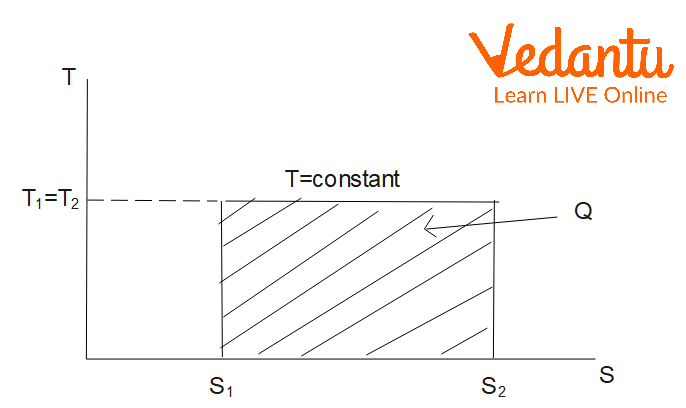Graph of Isothermal Process

4. Adiabatic Process: The adiabatic process is a thermodynamic process in which there is no heat transfer from in or out of the system. So, here $q=0$.

Therefore, from the entropy equation, we have

$\Delta S=\dfrac{q}{T}=0$

Entropy change in reversible adiabatic process therefore equals zero.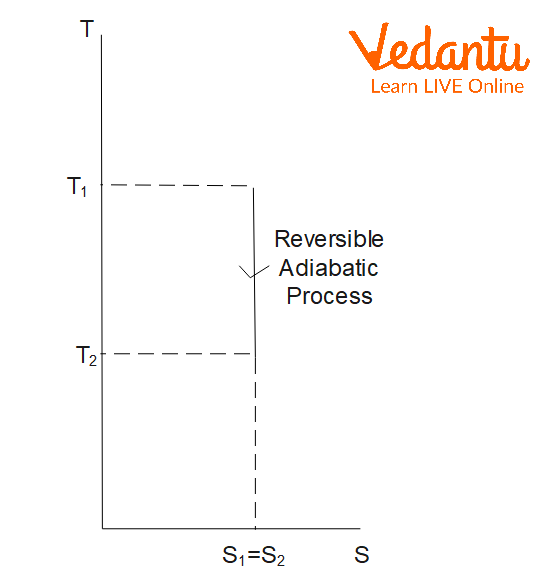5. Quasi-static Process: A quasi-static process is an infinitely slow process in which the system changes its variables (P,V,T) so slowly.

We can write the relation for entropy generation as

$dS=\dfrac{dQ}{T}+I$

Where dS denotes entropy change in the system.

The system can have a positive, negative, or zero entropy change.

6. Irreversible Adiabatic Process: As we know, the entropy change in an irreversible process will increase the total entropy of given surroundings. So, entropy change in irreversible adiabatic processes is also increasing. Frictional dissipation causes an alteration in entropy.

$dS\ge \dfrac{dQ}{T}$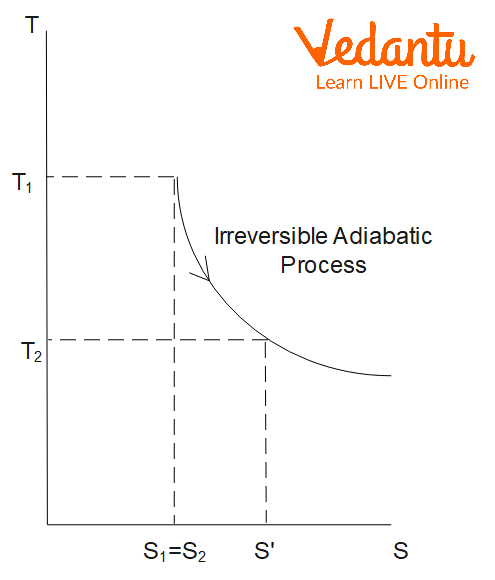## Examples of Entropy in Everyday Life

The entropy effects can be seen everywhere around us in this world; a few real-life examples of entropy are as mentioned below:

• Campfire

• Ice melting

• Salt or sugar dissolving

• Popcorn making

• Boiling water

• Drop of ink spreading out in a beaker of water

## Conclusion

In every process, the overall entropy of a system either rises or stays the same; it never falls. The entropy of the universe grows in irreversible process, such as the transmission of heat from a hot item to a cold object, whereas it remains constant in reversible processes. There are five types of thermodynamic processes, which include Isobaric process, Isochoric process, Isothermal process, Adiabatic process and Quasi-static process.

Last updated date: 15th Sep 2023
Total views: 125.1k
Views today: 3.25k

## FAQs on Entropy of Different Process for JEE

1. List the properties of entropy.

The properties of entropy are as follows:

• It is a thermodynamic function.

• It is a state function. It relies on how the system is functioning, not on the route taken.

• In the conventional state, it is represented by S°, however it is represented by S in this instance.

• Its CGS unit is cal/Kmol.

• As an extensive property, entropy grows or decreases in proportion to the size or extent of a system.

2. What causes entropy?

Numerous factors can affect a system's amount of entropy. Entropy rises in proportion to temperature.

• The molecules are excited and the amount of random activity increases as energy is added to a system.

• A rise in entropy occurs when a liquid turns into a gas.

• Entropy rises as a result of any chemical reaction that produces more gas molecules. An energetic chemical reaction that pours energy into a system would be one that introduces more gas molecules into the system. Entropy and atomic unpredictability increase as energy levels increase.